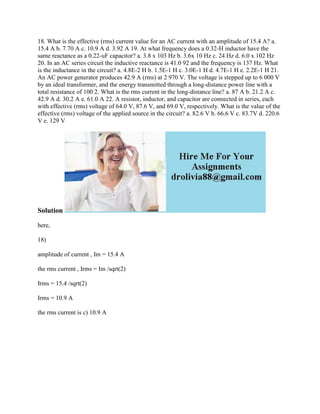Successfully reported this slideshow.

# 18- What is the effective (rms) current value for an AC current with a.docx×

# 18- What is the effective (rms) current value for an AC current with a.docx

18. What is the effective (rms) current value for an AC current with an amplitude of 15.4 A? a. 15.4 A b. 7.70 A c. 10.9 A d. 3.92 A 19. At what frequency does a 0.32-H inductor have the same reactance as a 0.22-uF capacitor? a. 3.8 x 103 Hz b. 3.6x 10 Hz c. 24 Hz d. 6.0 x 102 Hz 20. In an AC series circuit the inductive reactance is 41.0 92 and the frequency is 137 Hz. What is the inductance in the circuit? a. 4.8E-2 H b. 1.5E-1 H c. 3.0E-1 H d. 4.7E-1 H e. 2.2E-1 H 21. An AC power generator produces 42.9 A (rms) at 2 970 V. The voltage is stepped up to 6 000 V by an ideal transformer, and the energy transmitted through a long-distance power line with a total resistance of 100 2. What is the rms current in the long-distance line? a. 87 A b. 21.2 A c. 42.9 A d. 30.2 A e. 61.0 A 22. A resistor, inductor, and capacitor are connected in series, each with effective (rms) voltage of 64.0 V, 87.6 V, and 69.0 V, respectively. What is the value of the effective (rms) voltage of the applied source in the circuit? a. 82.6 V b. 66.6 V c. 83.7V d. 220.6 V e. 129 V
Solution
here,
18)
amplitude of current , Im = 15.4 A
the rms current , Irms = Im /sqrt(2)
Irms = 15.4 /sqrt(2)
Irms = 10.9 A
the rms current is c) 10.9 A
.

18. What is the effective (rms) current value for an AC current with an amplitude of 15.4 A? a. 15.4 A b. 7.70 A c. 10.9 A d. 3.92 A 19. At what frequency does a 0.32-H inductor have the same reactance as a 0.22-uF capacitor? a. 3.8 x 103 Hz b. 3.6x 10 Hz c. 24 Hz d. 6.0 x 102 Hz 20. In an AC series circuit the inductive reactance is 41.0 92 and the frequency is 137 Hz. What is the inductance in the circuit? a. 4.8E-2 H b. 1.5E-1 H c. 3.0E-1 H d. 4.7E-1 H e. 2.2E-1 H 21. An AC power generator produces 42.9 A (rms) at 2 970 V. The voltage is stepped up to 6 000 V by an ideal transformer, and the energy transmitted through a long-distance power line with a total resistance of 100 2. What is the rms current in the long-distance line? a. 87 A b. 21.2 A c. 42.9 A d. 30.2 A e. 61.0 A 22. A resistor, inductor, and capacitor are connected in series, each with effective (rms) voltage of 64.0 V, 87.6 V, and 69.0 V, respectively. What is the value of the effective (rms) voltage of the applied source in the circuit? a. 82.6 V b. 66.6 V c. 83.7V d. 220.6 V e. 129 V
Solution
here,
18)
amplitude of current , Im = 15.4 A
the rms current , Irms = Im /sqrt(2)
Irms = 15.4 /sqrt(2)
Irms = 10.9 A
the rms current is c) 10.9 A
.